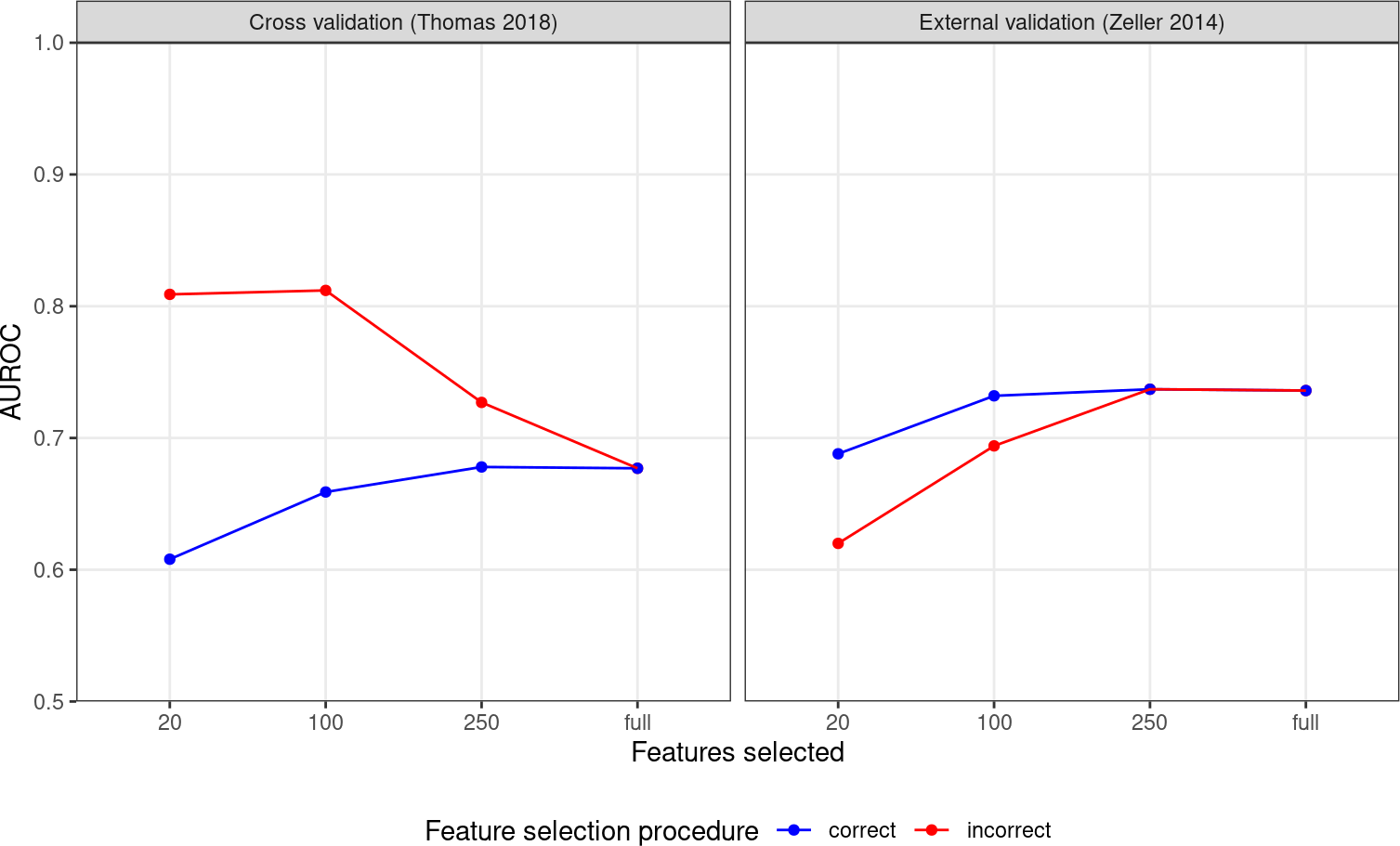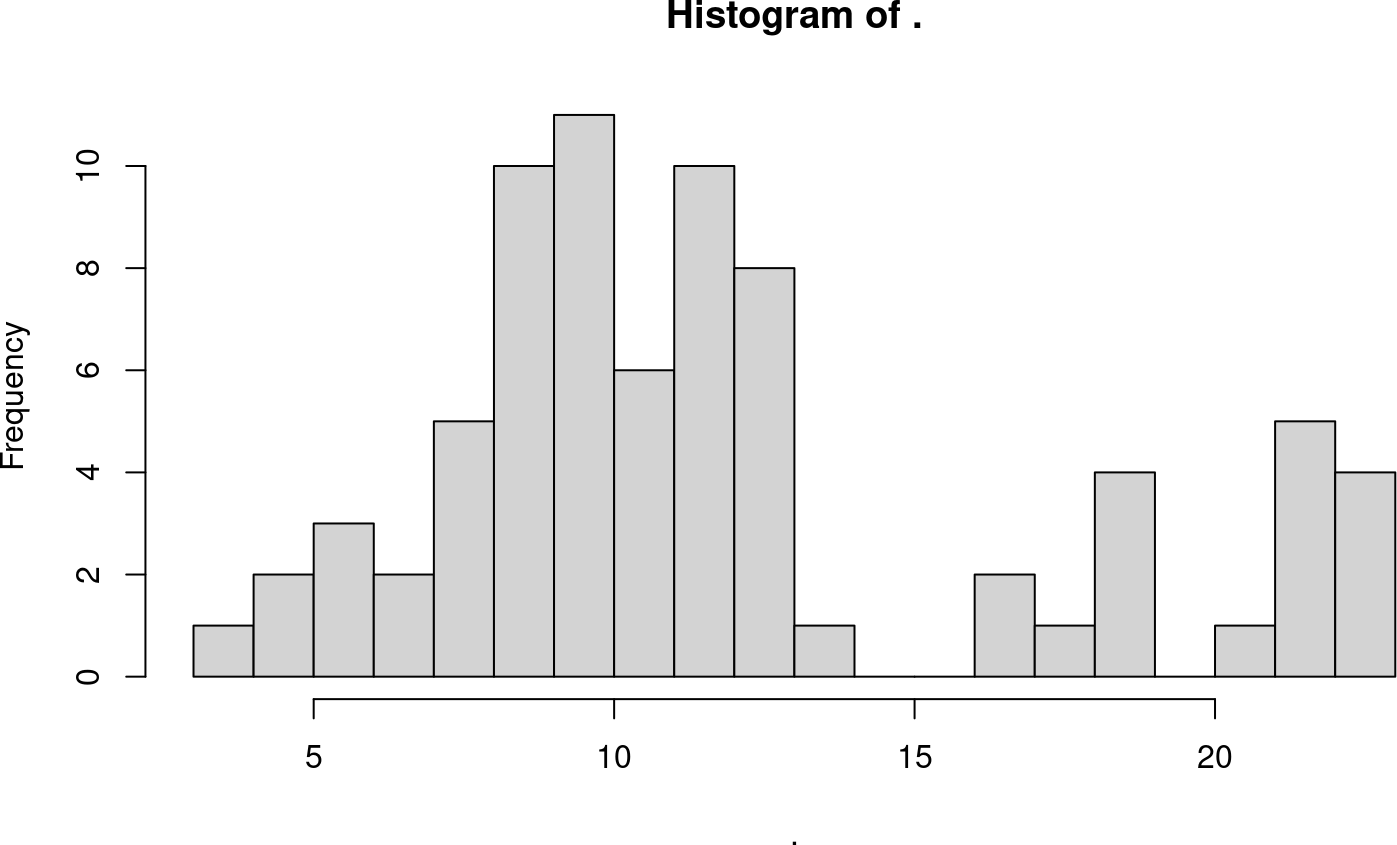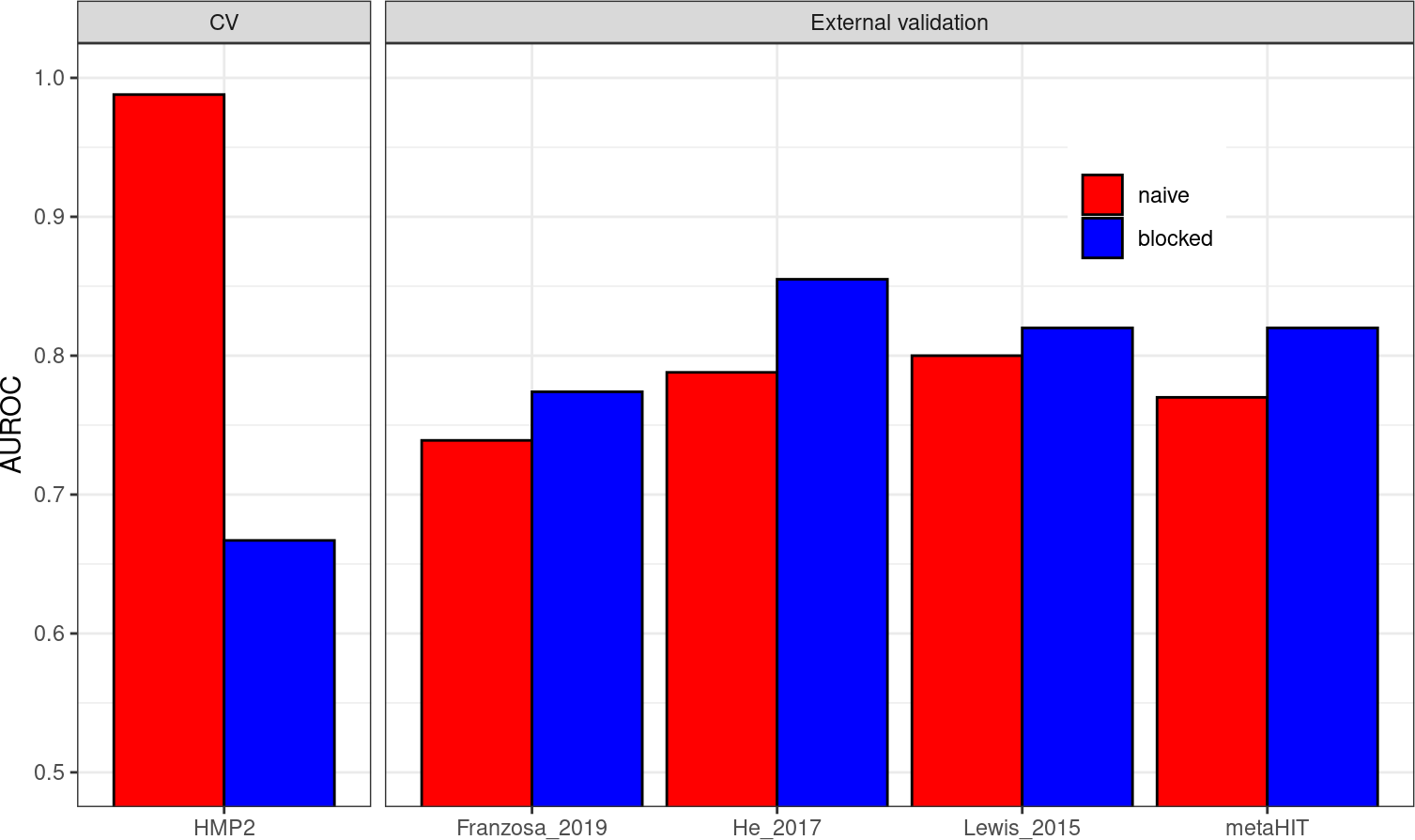# Contents

In this vignette, we want to explore two pitfalls for machine learning analysis that can lead to overly optimistic performance estimates.
When setting up cross-validation workflows, the main objective is usually to estimate how well a trained model would perform on external data, which is specifically important when considering biomarker discovery. However, more complex workflows involving feature selection or time-course data can be challenging to setup correctly. Incorrect workflows in which information leaks from the test to the training data can lead to overfitting and poor generalization to external datasets.
Here, we focus on supervised feature selection and the naive splitting of dependent data.

## 1.1 Setup

First, we load the packages needed to perform the analyses.

library("tidyverse")
library("SIAMCAT")

# 2 Supervised Feature Selection

Supervised feature selection means that the label information is taken into account before the cross-validation split. Within this procedure, the features are selected if they are associated with the label (for example after differential abundance testing), using the complete dataset for the calculation of feature association and leaving no data aside for unbiased model evaluation.
A correct way to perform feature selection would be to nest the selection step into the cross-validation procedure. That means that the calculation of feature association is performed for each training fold separately.

As an example, we are going to use two datasets of colorectal cancer (CRC) which are available through the curatedMetagenomicData package.
Since the model trainig procedure takes a long time, this vignette is not evaluated upon build of the package, but if you execute the code chunks for yourself, you should get similar results.

library("curatedMetagenomicData")

First, we are going to load the dataset from Thomas et al as training dataset.

x <- 'ThomasAM_2018a.metaphlan_bugs_list.stool'
feat.t <- curatedMetagenomicData(x=x, dryrun=FALSE)
feat.t <- feat.t[[x]]@assayData$exprs # clean up metaphlan profiles to contain only species-level abundances feat.t <- feat.t[grep(x=rownames(feat.t), pattern='s__'),] feat.t <- feat.t[grep(x=rownames(feat.t),pattern='t__', invert = TRUE),] stopifnot(all(colSums(feat.t) != 0)) feat.t <- t(t(feat.t)/100) As an external dataset, we are going to use the data from Zeller et al.. x <- 'ZellerG_2014.metaphlan_bugs_list.stool' feat.z <- curatedMetagenomicData(x=x, dryrun=FALSE) feat.z <- feat.z[[x]]@assayData$exprs
# clean up metaphlan profiles to contain only species-level abundances
feat.z <- feat.z[grep(x=rownames(feat.z), pattern='s__'),]
feat.z <- feat.z[grep(x=rownames(feat.z),pattern='t__', invert = TRUE),]
stopifnot(all(colSums(feat.z) != 0))
feat.z <- t(t(feat.z)/100)

We can also extract the corresponding metadata from the combined_metadata object which is part of the curatedMetagenomicData package.

meta.t <- combined_metadata %>%
filter(dataset_name == 'ThomasAM_2018a') %>%
filter(study_condition %in% c('control', 'CRC'))
rownames(meta.t) <- meta.t$sampleID meta.z <- combined_metadata %>% filter(dataset_name == 'ZellerG_2014') %>% filter(study_condition %in% c('control', 'CRC')) rownames(meta.z) <- meta.z$sampleID

The MetaPhlAn2 profiler used for the profiles outputs only species which are present in the dataset. Therefore, we can have the case that there are species in the matrix for ThomasAM_2018 which are not present in the matrix for ZellerG_2014 and vice verse. In order to use them as training and external test set for SIAMCAT, we have to first make sure that the set of features for both datasets overlap completely (see also the Holdout Testing with SIAMCAT vignette).

species.union <- union(rownames(feat.t), rownames(feat.z))
# add Zeller_2014-only species to the Thomas_2018 matrix
feat.t <- rbind(feat.t,

# add Thomas_2018-only species to the Zeller_2014 matrix
feat.z <- rbind(feat.z,
dimnames = list(add.species, colnames(feat.z))))

Now, we are ready to start the model training process. For this, we chose three different feature selection cutoffs and prepare a tibble to hold the results:

fs.cutoff <- c(20, 100, 250)

auroc.all <- tibble(cutoff=character(0), type=character(0),
study.test=character(0), AUC=double(0))

## 2.2 Train Model without Feature Selection

First, we will train a model without any feature selection, using all the available features. We add it to the results matrix twice (both with correct and incorrect) for easier plotting later.

sc.obj.t <- siamcat(feat=feat.t, meta=meta.t,
label='study_condition', case='CRC')
sc.obj.t <- filter.features(sc.obj.t, filter.method = 'prevalence',
cutoff = 0.01)
sc.obj.t <- normalize.features(sc.obj.t,
norm.method = 'log.std',
norm.param=list(log.n0=1e-05,
sd.min.q=0))
sc.obj.t <- create.data.split(sc.obj.t,
num.folds = 10, num.resample = 10)
sc.obj.t <- train.model(sc.obj.t, method='lasso')
sc.obj.t <- make.predictions(sc.obj.t)
sc.obj.t <- evaluate.predictions(sc.obj.t)

auroc.all <- auroc.all %>%
study.test='Thomas_2018',
AUC=as.numeric(sc.obj.t@eval_data$auroc)) %>% add_row(cutoff='full', type='incorrect', study.test='Thomas_2018', AUC=as.numeric(sc.obj.t@eval_data$auroc)) 

We then also apply the model to the external dataset and record the generalization to another dataset:

sc.obj.z <- siamcat(feat=feat.z, meta=meta.z,
label='study_condition', case='CRC')
sc.obj.z <- make.predictions(sc.obj.t, sc.obj.z)
sc.obj.z <- evaluate.predictions(sc.obj.z)
auroc.all <- auroc.all %>%
study.test='Zeller_2014',
AUC=as.numeric(sc.obj.z@eval_data$auroc)) %>% add_row(cutoff='full', type='incorrect', study.test='Zeller_2014', AUC=as.numeric(sc.obj.z@eval_data$auroc)) 

## 2.3 Incorrect Procedure: Train with Supervised Feature Selection

For the incorrect feature selection procedure, we can test the features for differential abundance, using the complete dataset, and then chose the top associated features.

sc.obj.t <- check.associations(sc.obj.t, detect.lim = 1e-05,
fn.plot = 'assoc_plot.pdf')
mat.assoc <- associations(sc.obj.t)
mat.assoc$species <- rownames(mat.assoc) # sort by p-value mat.assoc <- mat.assoc %>% as_tibble() %>% arrange(p.val) Based on the P values from the check.association function, we now chose X number of features on which to train the model. for (x in fs.cutoff){ # select x number of features based on p-value ranking feat.train.red <- feat.t[mat.assoc %>% slice(seq_len(x)) %>% pull(species),] sc.obj.t.fs <- siamcat(feat=feat.train.red, meta=meta.t, label='study_condition', case='CRC') # normalize the features without filtering sc.obj.t.fs <- normalize.features(sc.obj.t.fs, norm.method = 'log.std', norm.param=list(log.n0=1e-05, sd.min.q=0), feature.type = 'original') # take the same cross validation split as before data_split(sc.obj.t.fs) <- data_split(sc.obj.t) # train sc.obj.t.fs <- train.model(sc.obj.t.fs, method = 'lasso') # make predictions sc.obj.t.fs <- make.predictions(sc.obj.t.fs) # evaluate predictions and record the result sc.obj.t.fs <- evaluate.predictions(sc.obj.t.fs) auroc.all <- auroc.all %>% add_row(cutoff=as.character(x), type='incorrect', study.test='Thomas_2018', AUC=as.numeric(sc.obj.t.fs@eval_data$auroc))
# apply to the external dataset and record the result
sc.obj.z <- siamcat(feat=feat.z, meta=meta.z,
label='study_condition', case='CRC')
sc.obj.z <- make.predictions(sc.obj.t.fs, sc.obj.z)
sc.obj.z <- evaluate.predictions(sc.obj.z)
auroc.all <- auroc.all %>%
study.test='Zeller_2014',
AUC=as.numeric(sc.obj.z@eval_data$auroc)) } ## 2.4 Correct Procedure: Train with Nested Feature Selection Feature selection can be performed correctly if it is nested within the cross-validation procedure. We can do it using SIAMCAT by specifying the perform.fs parameter in the train.model function. for (x in fs.cutoff){ # train using the original SIAMCAT object # with correct version of feature selection sc.obj.t.fs <- train.model(sc.obj.t, method = 'lasso', perform.fs = TRUE, param.fs = list(thres.fs = x, method.fs = "AUC", direction='absolute')) # make predictions sc.obj.t.fs <- make.predictions(sc.obj.t.fs) # evaluate predictions and record the result sc.obj.t.fs <- evaluate.predictions(sc.obj.t.fs) auroc.all <- auroc.all %>% add_row(cutoff=as.character(x), type='correct', study.test='Thomas_2018', AUC=as.numeric(sc.obj.t.fs@eval_data$auroc))
# apply to the external dataset and record the result
sc.obj.z <- siamcat(feat=feat.z, meta=meta.z,
label='study_condition', case='CRC')
sc.obj.z <- make.predictions(sc.obj.t.fs, sc.obj.z)
sc.obj.z <- evaluate.predictions(sc.obj.z)
auroc.all <- auroc.all %>%
study.test='Zeller_2014',
AUC=as.numeric(sc.obj.z@eval_data$auroc)) } ## 2.5 Plot the Results Now, we can plot the resulting performance estimates for the cross-validation and the external validation as well: auroc.all %>% # facetting for plotting mutate(split=case_when(study.test=="Thomas_2018"~ 'Cross validation (Thomas 2018)', TRUE~"External validation (Zeller 2014)")) %>% # convert to factor to enforce ordering mutate(cutoff=factor(cutoff, levels = c(fs.cutoff, 'full'))) %>% ggplot(aes(x=cutoff, y=AUC, col=type)) + geom_point() + geom_line(aes(group=type)) + facet_grid(~split) + scale_y_continuous(limits = c(0.5, 1), expand = c(0,0)) + xlab('Features selected') + ylab('AUROC') + theme_bw() + scale_colour_manual(values = c('correct'='blue', 'incorrect'='red'), name='Feature selection procedure') + theme(panel.grid.minor = element_blank(), legend.position = 'bottom')As you can see, the incorrect feature selection procedure leads to inflated AUROC values but lower generalization to a truly external dataset, especially when very few features were selected. In contrast, the correct procedure gives a lower cross-validation results but a better estimation for how the model would perform on external data. # 3 Naive Splitting of Dependent Data Another issue in machine learning workflows can occur when samples are not independent. For example, microbiome samples taken from the same individual at different time points are usually more similar to each other than to samples from other individuals. If these samples are split randomly in a naive cross-validation procedure, the case could arise that samples from the same individual will end up in the training and the test fold. In this case, the model would learn to generalize across time-points for the same individual compared to the desired model that should learn to distinguish the label across individuals. To avoid this issue, dependent measurements need to be blocked during cross-validation, to ensure that samples within the same block will stay in the same fold (for training and testing). ## 3.1 Load the Data As an example, we are going to use several datasets of Crohn’s disease (CD) which are available through the EMBL cluster. The data have already been filtered and cleaned. Since the model training would take again quite a long time, this part of the vignette is not evaluated upon building of the package, but you should be able to execute it yourself. data.location <- 'https://www.embl.de/download/zeller/' # metadata meta.all <- read_tsv(paste0(data.location, 'CD_meta/meta_all.tsv')) ## ## ── Column specification ──────────────────────────────────────────────────────── ## cols( ## Sample_ID = col_character(), ## Group = col_character(), ## Library_Size = col_double(), ## Individual_ID = col_character(), ## Timepoint = col_double(), ## Study = col_character() ## ) # features feat.motus <- read.table(paste0(data.location, 'CD_meta/feat_rel_filt.tsv'), sep='\t', stringsAsFactors = FALSE, check.names = FALSE) When we look at the number of samples and number of individuals, we see that that there are several samples per individual for example in the HMP2 study. x <- meta.all %>% group_by(Study, Group) %>% summarise(n.all=n(), .groups='drop') y <- meta.all %>% select(Study, Group, Individual_ID) %>% distinct() %>% group_by(Study, Group) %>% summarize(n.indi=n(), .groups='drop') full_join(x,y) ## Joining, by = c("Study", "Group") ## # A tibble: 10 x 4 ## Study Group n.all n.indi ## <chr> <chr> <int> <int> ## 1 Franzosa_2019 CD 88 88 ## 2 Franzosa_2019 CTR 56 56 ## 3 HMP2 CD 583 50 ## 4 HMP2 CTR 357 26 ## 5 He_2017 CD 49 49 ## 6 He_2017 CTR 53 53 ## 7 Lewis_2015 CD 294 85 ## 8 Lewis_2015 CTR 25 25 ## 9 metaHIT CD 21 13 ## 10 metaHIT CTR 71 59 Therefore, we are going to train a model on the HMP2 study. However, the number of samples per individual varies quite a lot across samples, therefore we want to randomly select a set of 5 samples per individual: meta.all %>% filter(Study=='HMP2') %>% group_by(Individual_ID) %>% summarise(n=n(), .groups='drop') %>% pull(n) %>% hist(20)# sample 5 samples per individual meta.train <- meta.all %>% filter(Study=='HMP2') %>% group_by(Individual_ID) %>% sample_n(5, replace = TRUE) %>% distinct() %>% as.data.frame() rownames(meta.train) <- meta.train$Sample_ID

For evaluation, we only want a single sample per individual, therefore we can create a new matrix removing repeated samples for the other studies:

meta.ind <- meta.all %>%
group_by(Individual_ID) %>%
filter(Timepoint==min(Timepoint)) %>%
ungroup()

Lastly, we can already create a tibble to hold the resulting AUROC values:

auroc.all <- tibble(type=character(0), study.test=character(0),
AUC=double(0))

## 3.2 Train with Naive Cross-validation

The naive way to split samples for cross-validation does not take into account the dependency between samples. Therefore, the pipeline would look basically like this:

sc.obj <- siamcat(feat=feat.motus, meta=meta.train,
label='Group', case='CD')
sc.obj <- normalize.features(sc.obj, norm.method = 'log.std',
norm.param=list(log.n0=1e-05,
sd.min.q=1),
feature.type = 'original')
sc.obj.naive <- create.data.split(sc.obj, num.folds = 10, num.resample = 10)
sc.obj.naive <- train.model(sc.obj.naive, method='lasso')
sc.obj.naive <- make.predictions(sc.obj.naive)
sc.obj.naive <- evaluate.predictions(sc.obj.naive)
auroc.all <- auroc.all %>%

## 3.4 Apply to External Datasets

Now, we can apply both models to external datasets and record the resulting accuracy:

for (i in setdiff(unique(meta.all$Study), 'HMP2')){ meta.test <- meta.ind %>% filter(Study==i) %>% as.data.frame() rownames(meta.test) <- meta.test$Sample_ID
# apply naive model
sc.obj.test <- siamcat(feat=feat.motus, meta=meta.test,
label='Group', case='CD')
sc.obj.test <- make.predictions(sc.obj.naive, sc.obj.test)
sc.obj.test <- evaluate.predictions(sc.obj.test)
auroc.all <- auroc.all %>%
AUC=as.numeric(eval_data(sc.obj.test)$auroc)) # apply blocked model sc.obj.test <- siamcat(feat=feat.motus, meta=meta.test, label='Group', case='CD') sc.obj.test <- make.predictions(sc.obj.block, sc.obj.test) sc.obj.test <- evaluate.predictions(sc.obj.test) auroc.all <- auroc.all %>% add_row(type='blocked', study.test=i, AUC=as.numeric(eval_data(sc.obj.test)$auroc))
}

## 3.5 Plot the Results

Now, we can compare the resulting AUROC values between the two different approaches:

auroc.all %>%
# convert to factor to enforce ordering
mutate(type=factor(type, levels = c('naive', 'blocked'))) %>%
# facetting for plotting
mutate(CV=case_when(study.test=='HMP2'~'CV', TRUE~'External validation')) %>%

ggplot(aes(x=study.test, y=AUC, fill=type)) +
geom_bar(stat='identity', position = position_dodge(), col='black') +
theme_bw() +
coord_cartesian(ylim=c(0.5, 1)) +
scale_fill_manual(values=c('red', 'blue'), name='') +
facet_grid(~CV, space = 'free', scales = 'free') +
xlab('') + ylab('AUROC') +
theme(legend.position = c(0.8, 0.8))As you can see, the naive cross-validation procedure leads to a inflated performance estimation compared to the blocked cross-validation. However, when assessing generalization to truly external datasets, the blocked procedure results in better performance.

# 4 Session Info

sessionInfo()
## R version 4.1.0 (2021-05-18)
## Platform: x86_64-pc-linux-gnu (64-bit)
## Running under: Ubuntu 20.04.2 LTS
##
## Matrix products: default
## BLAS:   /home/biocbuild/bbs-3.13-bioc/R/lib/libRblas.so
## LAPACK: /home/biocbuild/bbs-3.13-bioc/R/lib/libRlapack.so
##
## locale:
##   LC_CTYPE=en_US.UTF-8       LC_NUMERIC=C
##   LC_TIME=en_GB              LC_COLLATE=C
##   LC_MONETARY=en_US.UTF-8    LC_MESSAGES=en_US.UTF-8
##   LC_PAPER=en_US.UTF-8       LC_NAME=C
##  LC_MEASUREMENT=en_US.UTF-8 LC_IDENTIFICATION=C
##
## attached base packages:
##  stats     graphics  grDevices utils     datasets  methods   base
##
## other attached packages:
##   ggpubr_0.4.0      SIAMCAT_1.12.0    phyloseq_1.36.0   mlr_2.19.0
##   ParamHelpers_1.14 forcats_0.5.1     stringr_1.4.0     dplyr_1.0.6
##   purrr_0.3.4       readr_1.4.0       tidyr_1.1.3       tibble_3.1.2
##  ggplot2_3.3.3     tidyverse_1.3.1   BiocStyle_2.20.0
##
## loaded via a namespace (and not attached):
##    corrplot_0.88          plyr_1.8.6             igraph_1.2.6
##    splines_4.1.0          GenomeInfoDb_1.28.0    gridBase_0.4-7
##   digest_0.6.27          foreach_1.5.1          htmltools_0.5.1.1
##   magick_2.7.2           fansi_0.4.2            magrittr_2.0.1
##   checkmate_2.0.0        BBmisc_1.11            cluster_2.1.2
##   openxlsx_4.2.3         Biostrings_2.60.0      modelr_0.1.8
##   matrixStats_0.58.0     prettyunits_1.1.1      colorspace_2.0-1
##   rvest_1.0.0            haven_2.4.1            xfun_0.23
##   crayon_1.4.1           RCurl_1.98-1.3         jsonlite_1.7.2
##   survival_3.2-11        iterators_1.0.13       ape_5.5
##   glue_1.4.2             gtable_0.3.0           zlibbioc_1.38.0
##   XVector_0.32.0         car_3.0-10             Rhdf5lib_1.14.0
##   shape_1.4.6            BiocGenerics_0.38.0    abind_1.4-5
##   scales_1.1.1           infotheo_1.2.0         DBI_1.1.1
##   rstatix_0.7.0          Rcpp_1.0.6             progress_1.2.2
##   foreign_0.8-81         stats4_4.1.0           glmnet_4.1-1
##   httr_1.4.2             RColorBrewer_1.1-2     ellipsis_0.3.2
##   XML_3.99-0.6           pkgconfig_2.0.3        farver_2.1.0
##   sass_0.4.0             dbplyr_2.1.1           utf8_1.2.1
##   tidyselect_1.1.1       labeling_0.4.2         rlang_0.4.11
##   reshape2_1.4.4         PRROC_1.3.1            munsell_0.5.0
##   cellranger_1.1.0       tools_4.1.0            cli_2.5.0
##   evaluate_0.14          biomformat_1.20.0      yaml_2.2.1
##   knitr_1.33             fs_1.5.0               zip_2.1.1
##   beanplot_1.2           nlme_3.1-152           xml2_1.3.2
##   compiler_4.1.0         rstudioapi_0.13        curl_4.3.1
##   ggsignif_0.6.1         reprex_2.0.0           bslib_0.2.5.1
##   stringi_1.6.2          highr_0.9              ps_1.6.0
##   lattice_0.20-44        Matrix_1.3-3           vegan_2.5-7
##   permute_0.9-5          multtest_2.48.0        vctrs_0.3.8
##   pillar_1.6.1           lifecycle_1.0.0        rhdf5filters_1.4.0
##  BiocManager_1.30.15    jquerylib_0.1.4        LiblineaR_2.10-12
##  data.table_1.14.0      bitops_1.0-7           R6_2.5.0
##  bookdown_0.22          gridExtra_2.3          rio_0.5.26
##  IRanges_2.26.0         codetools_0.2-18       MASS_7.3-54
##  assertthat_0.2.1       rhdf5_2.36.0           withr_2.4.2
##  S4Vectors_0.30.0       GenomeInfoDbData_1.2.6 mgcv_1.8-35
##  parallel_4.1.0         hms_1.1.0              grid_4.1.0
##  rmarkdown_2.8          carData_3.0-4          parallelMap_1.5.0
##  pROC_1.17.0.1          Biobase_2.52.0         lubridate_1.7.10